• 提出了一种基于隐朴素贝叶斯（hidden nave Bayesian，HNB）模型的用户关系推荐算法。该算法通过分析属性之间的依赖性对问题建模，从中度量共邻用户之间关系对推荐用户对之间的贡献和影响，然后对所有候选推荐关系...社会网络
• 针对传统朴素贝叶斯算法对高维复杂的入侵行为检测效率低下的状况，提出一种基于粒子群的加权朴素贝叶斯入侵检测模型模型首先用粗糙集理论对样本属性特征集进行约简，再利用改进的粒子群算法优化加权朴素贝叶斯算法...
• 针对朴素贝叶斯分类器在分类过程中不同类别的同一特征量之间由于存在相似性，易导致误分类的现象，提出基于引力模型朴素贝叶斯分类算法。提出以引力公式中距离变量的平方作为相似距离，应用引力模型来刻画特征与其...分类算法 遥感图像
• 论文研究-扩展的树增强朴素贝叶斯网络信用评估模型.pdf,
• 树增强朴素贝叶斯模型通过放松条件属性独立来改进贝叶斯模型，结构学习效率较高且简单。然而在一些实际实验测试中，树增强朴素贝叶斯分类模型的分类精确性和失误率的效果却不好。因此，设计了加权平均的树增强朴素...
• 基于朴素贝叶斯分类器的LTE网络数据面故障诊断模型，张家望，刘培植，在本篇文章中，我们提出一种利用朴素贝叶斯分类器针对LTE网络数据面中发生的网络故障进行自动化分析定位的方法。针对LTE网络中TCP下通信与信息系统
• 首先在朴素贝叶斯模型中引入粗糙集技术对数据集进行属性约简，获取最优属性子集；然后在此基础上以最大化数据集的对数条件似然估计为标准对条件属性设定（近似）最优权值，进而提出一种新型加权粗糙朴素贝叶斯模型。...决策表 属性约简 垃圾邮件过滤
• 由于多年来互联网用户数量的大幅增长，社交媒体用户数量也出现了大幅增长。... 我们观察到像朴素贝叶斯这样的生成模型通常比 SVM 和决策树等判别模型在语料库较小的微博网站上执行情感分析任务时表现更好。
• 针对传统朴素贝叶斯分类模型在入侵取证中存在的特征项冗余问题，以及没有考虑入侵行为所涉及的数据属性间的差别问题，提出一种基于改进的属性加权朴素贝叶斯分类方法。用一种改进的基于特征冗余度的信息增益算法对...
• 本文在朴素贝叶斯模型和EM（expectation maximization）算法的基础上，提出了针对软件工作量数据缺失的处理方法.首先，本文介绍了数据缺失的内在机理；然后，本文提出了基于朴素贝叶斯和EM算法的软件工作量预测的...
• amp;rep=rep1&amp;type=pdf综述性论文，讲述基本概念，介绍使用实验来验证可以达成提高多大的提高。目前决策树已十分成熟，业内论文主要... 朴素贝叶斯算法在很多分类器项目上表现出惊异的准确性，即使在依赖的...
       http://citeseerx.ist.psu.edu/viewdoc/download?doi=10.1.1.462.9093&rep=rep1&type=pdf综述性论文，讲述基本概念，介绍使用实验来验证可以达成提高多大的提高。目前决策树已十分成熟，业内论文主要也针对新的应用场景，以及多模型融合。 朴素贝叶斯算法在很多分类器项目上表现出惊异的准确性，即使在依赖的条件独立性假设条件被违反的情况下。但这主要是在一些数据量较小的项目上。在一些数据量很大的项目中，贝叶斯的表现不如决策树的准确率高。针对这个问题，我们提出了一种新的算法：NB树，混合了决策树分类器跟贝叶斯分类器。决策树包含单变量的节点分裂为常规决策树，叶节点包含贝叶斯分类器。这种方法在比两种原始方案表现更好的情况下（特别是在数据量较的情况下）保留了朴素贝叶斯与决策树的解释性。简介朴素贝叶斯算法对不相关特性具有很好的鲁棒性，缺点是要求做强独立假设，如果假设被违反，可实现的渐近线会很早靠近，准确性不会随着数据规模的增大增大。决策树缺点在于基于递归划分的当前归纳方法存在着分割问题，每次分割完成后数据集越来越小，最后只能依赖很小的数据来做决定。两者都有很好的分类可读性。NB树结合了两者的优点，通过决策树分割数据后，代表一份数据片段的叶节点，被贝叶斯分类器适用。接下来在算法归纳中会显示条件独立假设会被满足。NBTree：融合算法输入:一组标记的实例。输出:在叶节点上具有naive-bayes分类器的决策树。
展开全文• 然后利用扩展逻辑回归的朴素贝叶斯算法对聚类结果建立预测模型；最后使用增量式更新的方法，在不全部重新训练模型的基础上，对模型进行局部修改。实验结果表明，该算法较传统的协同过滤算法在一定程度上克服了数据...推荐算法
• 在印度，第四大主要疾病之一是甲状腺疾病，它对社会构成严重威胁，因为家庭人口，气候，城市化，食物的变化增加了甲状腺激素模拟变化... 本文使用J48和朴素贝叶斯分类技术进行比较，以基于效率和准确性来充分利用算法。
• 朴素贝叶斯：生成式模型，条件独立 —&amp;amp;gt; 序列形式 隐马尔科夫模型 —&amp;amp;gt; 图形式 通用有向图模型 逻辑回归：判别式模型，条件不独立 —&amp;amp;gt; 序列形式 线性链条件随机场 —&...

一直在搞CNN/RNN，对传统的知识了解一直不够，今天恰好看一篇论文需要CRF的知识，就借机都学习一下

梗概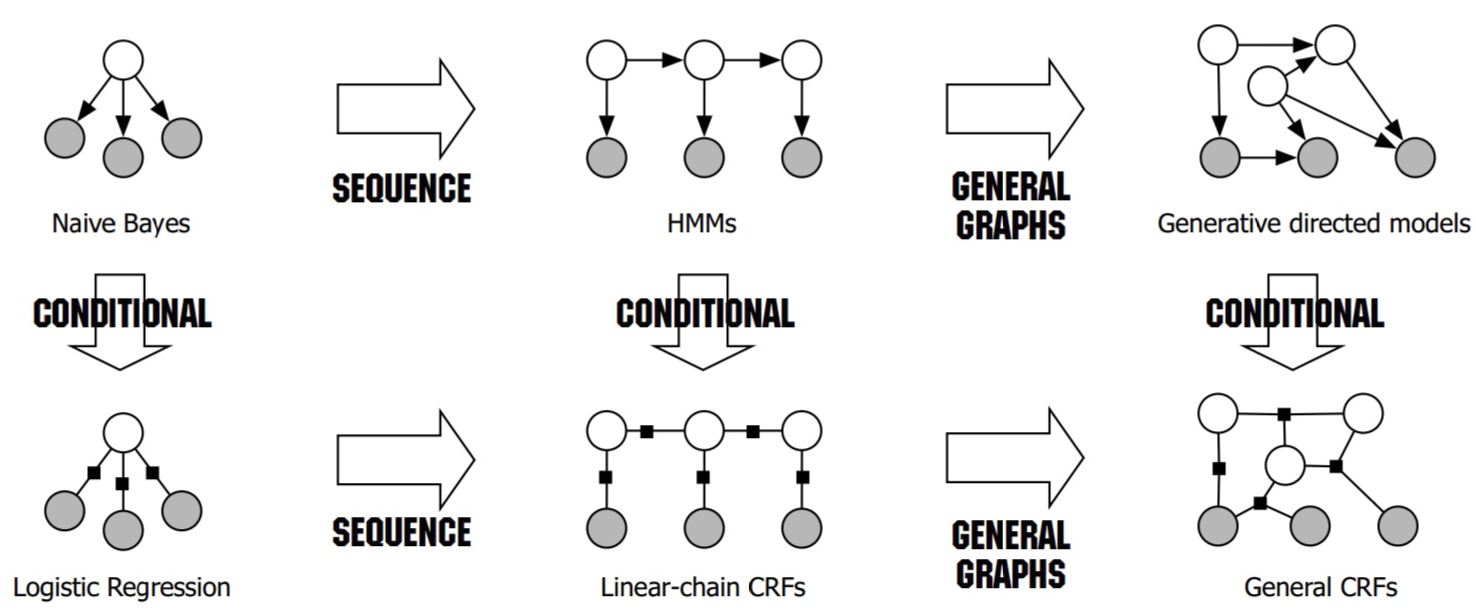朴素贝叶斯：生成式模型，条件独立 —> 序列形式 隐马尔科夫模型  —> 图形式 通用有向图模型
逻辑回归：判别式模型，条件不独立 —> 序列形式 线性链条件随机场 —> 序列形式 通用条件随机场

朴素贝叶斯(NB)

贝叶斯公式
P(Y|X)=P(X|Y)∗P(Y)P(X)$P\left(Y|X\right)=\frac{P\left(X|Y\right)\ast P\left(Y\right)}{P\left(X\right)}$ P(Y|X) = \frac {P(X|Y) * P(Y)} {P(X)}
一般形式:
P(Y|X1,X2,X3,...Xn)=P(X1,X2,X3,...Xn|Y)∗P(Y)P(X1,X2,X3,...Xn)$P\left(Y|{X}_{1},{X}_{2},{X}_{3},...{X}_{n}\right)=\frac{P\left({X}_{1},{X}_{2},{X}_{3},...{X}_{n}|Y\right)\ast P\left(Y\right)}{P\left({X}_{1},{X}_{2},{X}_{3},...{X}_{n}\right)}$P(Y|X_1, X_2, X_3, ...X_n) = \frac {P(X_1, X_2, X_3, ...X_n|Y) * P(Y)} {P(X_1, X_2, X_3, ...X_n)}
条件独立性假设: 特征之间互相独立，没有耦合，互不干扰。
P(X1,X2,X3,...Xn|Y)=P(X1|Y)∗P(X2|Y)∗P(X3|Y)∗...P(Xn|Y)$P\left({X}_{1},{X}_{2},{X}_{3},...{X}_{n}|Y\right)=P\left({X}_{1}|Y\right)\ast P\left({X}_{2}|Y\right)\ast P\left({X}_{3}|Y\right)\ast ...P\left({X}_{n}|Y\right)$P(X_1, X_2, X_3, ...X_n | Y) = P(X_1|Y) * P(X_2|Y) * P(X_3|Y) * ...P(X_n|Y)
===>
P(Y|X1,X2,X3,...Xn)=P(X1,X2,X3,...Xn|Y)∗P(Y)P(X1,X2,X3,...Xn)=[P(X1|Y)∗P(X2|Y)∗P(X3|Y)∗...P(Xn|Y)]∗P(Y)P(X1,X2,X3,...Xn)$P\left(Y|{X}_{1},{X}_{2},{X}_{3},...{X}_{n}\right)=\frac{P\left({X}_{1},{X}_{2},{X}_{3},...{X}_{n}|Y\right)\ast P\left(Y\right)}{P\left({X}_{1},{X}_{2},{X}_{3},...{X}_{n}\right)}=\frac{\left[P\left({X}_{1}|Y\right)\ast P\left({X}_{2}|Y\right)\ast P\left({X}_{3}|Y\right)\ast ...P\left({X}_{n}|Y\right)\right]\ast P\left(Y\right)}{P\left({X}_{1},{X}_{2},{X}_{3},...{X}_{n}\right)}$P(Y|X_1, X_2, X_3, ...X_n) = \frac {P(X_1, X_2, X_3, ...X_n|Y) * P(Y)} {P(X_1, X_2, X_3, ...X_n)} = \frac {[P(X_1|Y) * P(X_2|Y) * P(X_3|Y) * ...P(X_n|Y)] * P(Y)} {P(X_1, X_2, X_3, ...X_n)}

因为有条件独立假设，朴素贝叶斯可以不使用梯度下降，而直接通过统计每个特征的逻辑发生比来当做权重。
它是生成模型，实际上用作分类时比的是分子大小，即联合概率分布P(X,Y)，而P(X,Y)=P(X|Y)*P(Y), P(X|Y)和P(Y)都可由从训练数据里统计获得
P(Y|X)=P(X,Y)P(X)=P(X|Y)∗P(Y)P(X)$P\left(Y|X\right)=\frac{P\left(X,Y\right)}{P\left(X\right)}=\frac{P\left(X|Y\right)\ast P\left(Y\right)}{P\left(X\right)}$ P(Y|X) = \frac {P(X,Y)} {P(X)} = \frac {P(X|Y) * P(Y)} {P(X)}

逻辑回归(LR)

逻辑回归(Logistic Regression)与线性回归(Linear Regression)都是一种广义线性模型(generalized linear model)因此与线性回归有很多相同之处，去除Sigmoid映射函数的话，逻辑回归就是一个线性回归。可以说，逻辑回归是以线性回归为理论支持的，但是逻辑回归通过Sigmoid函数引入了非线性因素，因此可以轻松处理0/1分类问题。
注意逻辑回归和线性回归都是回归，但是线性回归就是用来回归，而是逻辑回归回归的是概率，是用来分类的，这是因为由于条件之间不独立，不能求出联合概率分布，只能回归后验概率，大于0.5即为yes，所以是判别模型
P(y|x)=g(wTx)=11+exp(−wTx)$P\left(y|x\right)=g\left({w}^{T}x\right)=\frac{1}{1+exp\left(-{w}^{T}x\right)}$ P(y|x) = g(w^Tx) =  \frac {1}{1+exp(-w^Tx)}
损失函数(逻辑回归就是BCE，二分类是逻辑回归，多分类就是softmax):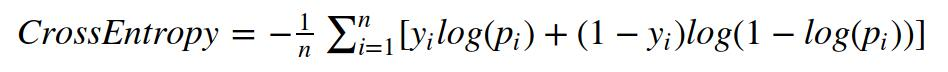线性回归中的代价函数看上去很好理解，但却不能用于逻辑回归，原因如下：由于g(x)是一个sigmoid函数，使用mse作为损失函数，会成为一个非凸函数，因此，我们需要另外找到一个不同的代价函数，它是凸函数，使得我们可以使用很好的算法，如梯度下降法，而且能保证找到全局最小值。

从概率角度解释(最大似然估计)：
逻辑回归假设因变量 y 服从伯努利分布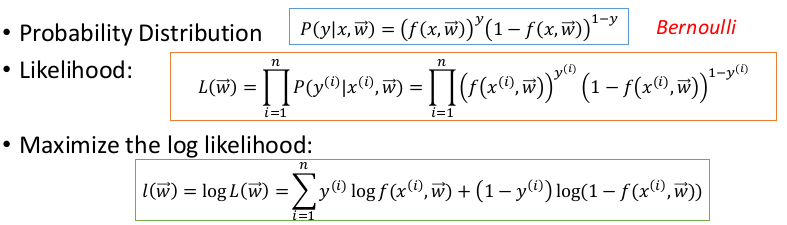线性回归假设因变量 y 服从高斯分布 y=f(x,w)+ε$y=f\left(x,w\right)+\epsilon$$y=f(x,w)+ ε$, ε is a zero mean Gaussian with precision β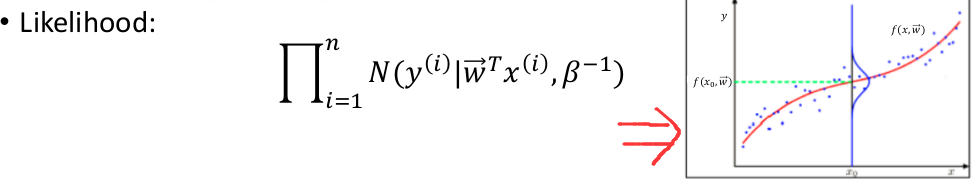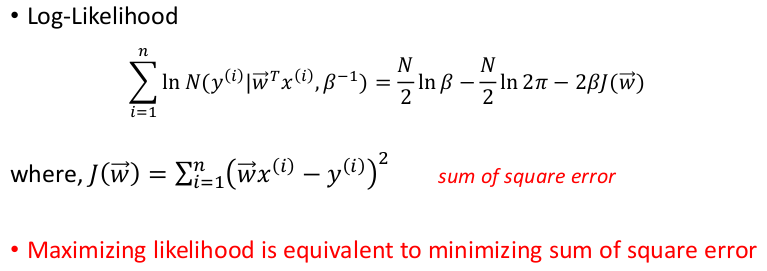线性回归的MSE损失函数加上L2正则化后，概率解释就是在MAP中假设权重W服从高斯分布了，这个更多解释搜索MLE(Maximum Likelihood Estimation)和MAP(Maximum A Posteriori)

隐马尔科夫模型(HMM)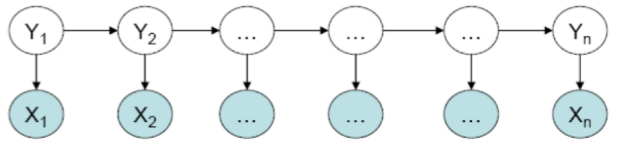HMM模型中存在两个假设：一是输出观察值之间严格独立，二是状态的转移过程中当前状态只与前一状态有关。
可以求出联合概率分布: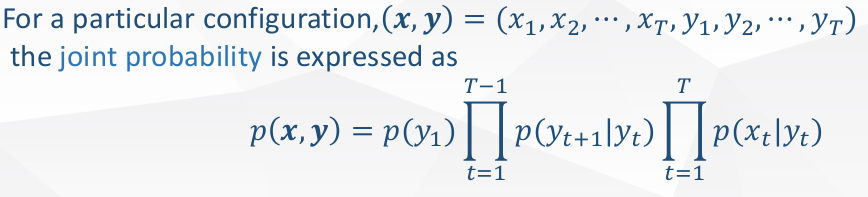例子参考李航老师的《统计机器学习方法》p173 例10.1：概率计算算法有有前向算法(α算法)和后向算法(β算法)

(线性链)条件随机场(CRF)

HMM模型中存在两个假设：一是输出观察值之间严格独立，二是状态的转移过程中当前状态只与前一状态有关。但实际上序列标注问题不仅和单个词相关，而且和观察序列的长度，单词的上下文，等等相关。MEMM解决了HMM输出独立性假设的问题。因为HMM只限定在了观测与状态之间的依赖，而MEMM引入自定义特征函数，不仅可以表达观测之间的依赖，还可表示当前观测与前后多个状态之间的复杂依赖。
这里由于去掉了独立性假设，所以不能给出联合概率分布，只能求后验概率，所以是判别模型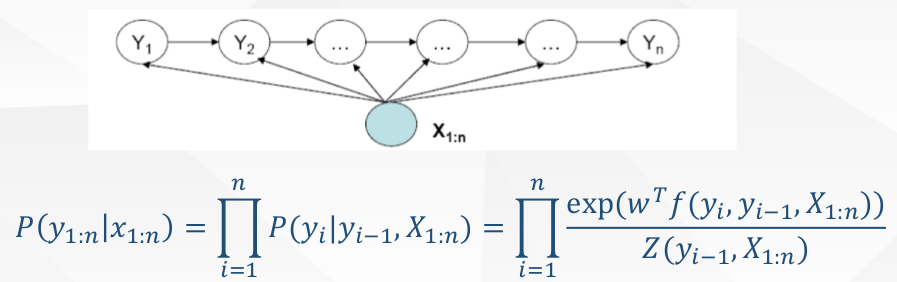但是MEMM存在标注偏置问题(Label Bias Problem)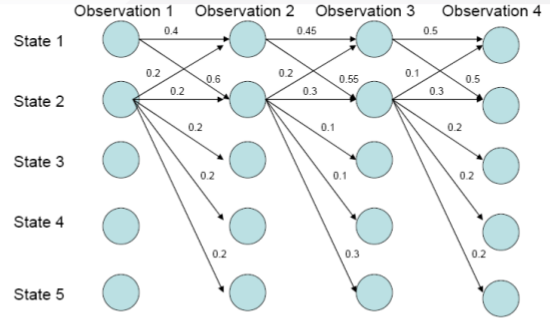实际上，在上图中，状态1偏向于转移到状态2，而状态2总倾向于停留在状态2。
而路径1-1-1-1的概率：0.4*0.45*0.5=0.09
而路径1-2-2-2的概率：0.6*\0.3*0.3=0.054
这是因为s1的转移状态很少，由于每一步的状态转移概率都要归一化，所以s1的转移概率都会被放大，而s2由于转移状态多，因此每一步转移概率归一化的时候都被平均分摊了(比如s2到s2在那个状态最大但由于s2的转移状态多，一分摊才为0.3，而s1到s2在它那个状态下概率最小但是由于转移状态少，不用分摊太多为0.4)。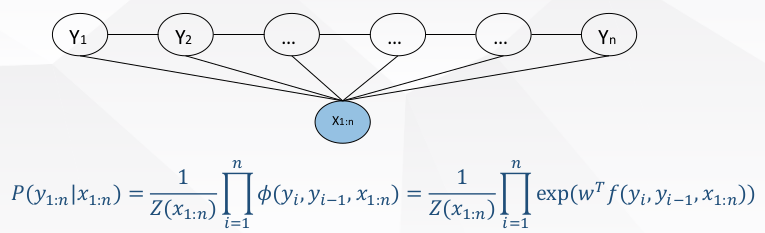CRF不仅解决了HMM输出独立性假设的问题，还解决了MEMM的标注偏置问题，MEMM容易陷入局部最优是因为只在局部做归一化，而CRF统计了全局概率，在做归一化时考虑了数据在全局的分布，而不是仅仅在局部归一化，这样就解决了MEMM中的标记偏置的问题。使得序列标注的解码变得最优解，和MEMM一样是判别模型

Coursera ML笔记 - 逻辑回归（Logistic Regression）
概率图模型体系：HMM、MEMM、CRF
朴素贝叶斯理论推导与三种常见模型
聊一聊机器学习的MLE和MAP：最大似然估计和最大后验估计
logistic回归和线性回归的区别
逻辑回归与朴素贝叶斯有什么区别？： 霍华德和陈默的回答
如何用简单易懂的例子解释条件随机场（CRF）模型？它和HMM有什么区别？：Scofield的回答
条件随机场(Conditional Random Field)简介
标注偏置问题(Label Bias Problem)和HMM、MEMM、CRF模型比较
概率图模型体系：HMM、MEMM、CRF

展开全文逻辑回归 条件随机场
• 同时还保证了当进行模型转换时，在CAD模型上必须作相应的几何改变，系统会同步变化，这样避免了传统的从CAD模型转换到VR模型转换时人工加入虚拟现实特殊信息，如光照设置、材质、纹理、行为这些刻画VR模型可视化特性...
• 使用Logistic回归模型进行中文文本分类，通过实验，比较和分析了不同的中文文本特征、不同的特征数目、不同文档集合的情况下，基于Logistic回归模型的分类器的性能。并将其与线性SVM文本分类器进行了比较，结果显示...
• 扩展了Rough集正区域和边界的...此外，在认知正区域的定义下，由于决策表的不相容性，在变精度模型下实现属性约简的增量处理是相当困难的，结合提出的高效算法，解决了这一问题。最后，仿真实验说明了算法的有效性。
• 分析了目前在垃圾邮件过滤中广泛应用的基于最小风险的朴素贝叶斯模型，提出了一种新的基于直线几何分割的朴素贝叶斯邮件过滤模型LGDNBF，定义了新的风险因子。新的风险因子对决策风险的描述更加精确，同时使得LGDNBF...垃圾邮件过滤
• 像你们大多数人一样，我花时间做饭，健身，编码和在周末阅读一些最新的研究论文。 当我注意到对数据科学的兴趣时，我开始考虑成为数据科学。 听起来真是太不可思议了，根据给定的数据，我们正在预测某天-今天的活动...
• / NLP学习/ 结合python学习自然语言处理 目录 论文实现
• 然后，最有希望的规则与朴素贝叶斯、相关向量机、贝叶斯模型平均、贝叶斯模型选择和贝叶斯正则化神经网络模型相结合。 就我们的结果而言，技术分析在外汇交易中具有价值，但利润空间很小。 另一方面，贝叶斯统计...
• 在这个模型中使用了两种构建的贝叶斯算法（朴素贝叶斯和贝叶斯网络），以及学生的问卷答案。 问卷由 62 个问题组成，涵盖了对学生表现影响最大的领域。 这些问题涉及健康、社交活动、人际关系和学业成绩。 问卷是...
• 引入不确定贝叶斯算法，将不确定数据的可能世界模型朴素贝叶斯分类模型结合起来，构建了不确定贝叶斯分类模型，从而有效刻画降雨量这一属性级不确定的属性，达到提高滑坡危险性预测精度的目的。通过实例验证了运用...
• 基于贝叶斯和规则的混合情感分析研究，王淼，孟洛明，朴素贝叶斯分类基于独立假设，对双重否定等词句不能准确分类。规则模型对网络新词等不能及时捕获，需要手动添加。本文在对各种分
• 贝叶斯网络是实现学习风格自动检测的重要手段，而TAN贝叶斯网络作为改进的朴素贝叶斯网络，具有更优的分类精度。以FSLSM模型为基础，提出了基于学习风格预设的TAN贝叶斯网络学习风格模型，通过挖掘学生的网络学习...
• 朴素贝叶斯分类器在处理垃圾邮件过滤任务时，往往存在数据稀疏问题。由于语料库中特征出现遵循Zipf定律，所以单纯依靠增加训练语料方式难以解决该问题。为克服数据稀疏问题，引入数据平滑算法计算贝叶斯模型中缺失...
• 在中医药临床个体化诊疗信息平台的基础上，使用中医证型的辨证相关因素，利用信息增益算法进行辨证属性选择，并分别采用朴素贝叶斯和强属性集贝叶斯网络算法建立了中医冠心病临床证型诊断模型。实验结果表明该分类...贝叶斯网络 数据挖掘
• 通过法国某装备生产企业的实例分析，实验结果证明在所有的贝叶斯网络分类器及传统的决策树C4.5分类器中，树型朴素贝叶斯分类器能够取得最好的分类效果，并为后续的维修资源配置及产品运行能力优化提供有效的理论支持...分类器
• 一组MATLAB脚本，用于实现我们提出的贝叶斯FQR模型（已调整或未调整），以及朴素的逐点贝叶斯分位数回归； R脚本实现了基于引导程序的方法，与我们在本文中提出的模型进行了比较； 复制纸上所有数字的代码； 该代码...
• 普适计算是信息空间与物理空间的融合,在这个融合的空间中人们可以随时随地和透明地获得数字化的服务。...根据朴素贝叶斯分类算法，提出了一种新的普适计算环境下的安全检测模型，并阐述了它的实现方法和特点。普适计算...